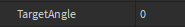# How set tank turret angle to where player's mouse is pointing at

I want setto where player’s mouse is pointing at. How to do it?
I tried this

``````local Players = game:GetService("Players")
local Player = Players.LocalPlayer
local Mouse = Player:GetMouse()

local RunService = game:GetService("RunService")
RunService.Heartbeat:Connect(function()
workspace.Maus.Turret.CylindricalConstraint.TargetAngle = Mouse.Hit.LookVector.X * 50
end)
``````

but it doesn’t rotate the tank’s turret to all positions to where player’s mouse is pointing at.

May I recommend setting the C1 or C0 of the Motor6D/Weld instead of the TargetAngle. To smooth it out use tweening.

Thanks, but the problem is that the tank’s turret won’t go any further than those red lines:

and I don’t know what’s causing it.

I’ve never worked with this object before but If I were to guess you’d probably have to find the angle between your Mouse’s LookVector relative to the Cylinder, and the Cylinder’s current LookVector, if that makes sense? Idk someone correct me

This might look something like this

``````local cylinderCurrentLookVector = whatever
local mouseCurrentLookVector = Mouse.Hit.LookVector
local dotProduct = cylinderCurrentLookVector:Dot(mouseCurrentLookVector)
``````

Looking at this image, we know that the dot product is equal to
the product of the magnitude of both vectors, then multiplied by the cosine of the angle(theta)therefore:

``````-- our dotProduct formula
dotProduct = cos(theta) * (cylinderCurrentLookVector.Magnitude * mouseCurrentLookVector.Magnitude)

-- transpose so that cos(theta) is the subject
-cos(theta) * dotProduct =  (cylinderCurrentLookVector.Magnitude * mouseCurrentLookVector.Magnitude)
-cos(theta) =  (cylinderCurrentLookVector.Magnitude * mouseCurrentLookVector.Magnitude) / dotProduct

-- now that we're trying to find theta, aka our angle and not the ratio we can go ahead and find the
-- inverse cosine of our formula to get theta

theta = -math.acos((cylinderCurrentLookVector.Magnitude * mouseCurrentLookVector.Magnitude) / dotProduct)

``````

This should be your overall formula to finding the angle between the two vectors, I’m not too sure how the CylindricalConstraint works like I said, but I’m guessing if you plug theta into the TargetAngle it may rotate the Cosntraint on the axis

1 Like

One question tho, how do you get LookVector of Cylinder, cause it’s not a part or a instance that has CFrame in it.

That’s the thing I was wondering about, is it possible for you to send a placefile for me so I can experiment a bit or I’ll just go make a new one and see

That is incorrect. Cylinder is simply a shape of Parts. Parts have a CFrame property and CFrames have a LookVector property.

1 Like

A cylinder falls under the class of BasePart in which has a CFrame value which you can use to get the LookVector of the CFrame.

Guys, he meant the CylindricalConstraint, not the actual BasePart lmao

1 Like

tank.rbxl (141.3 KB)